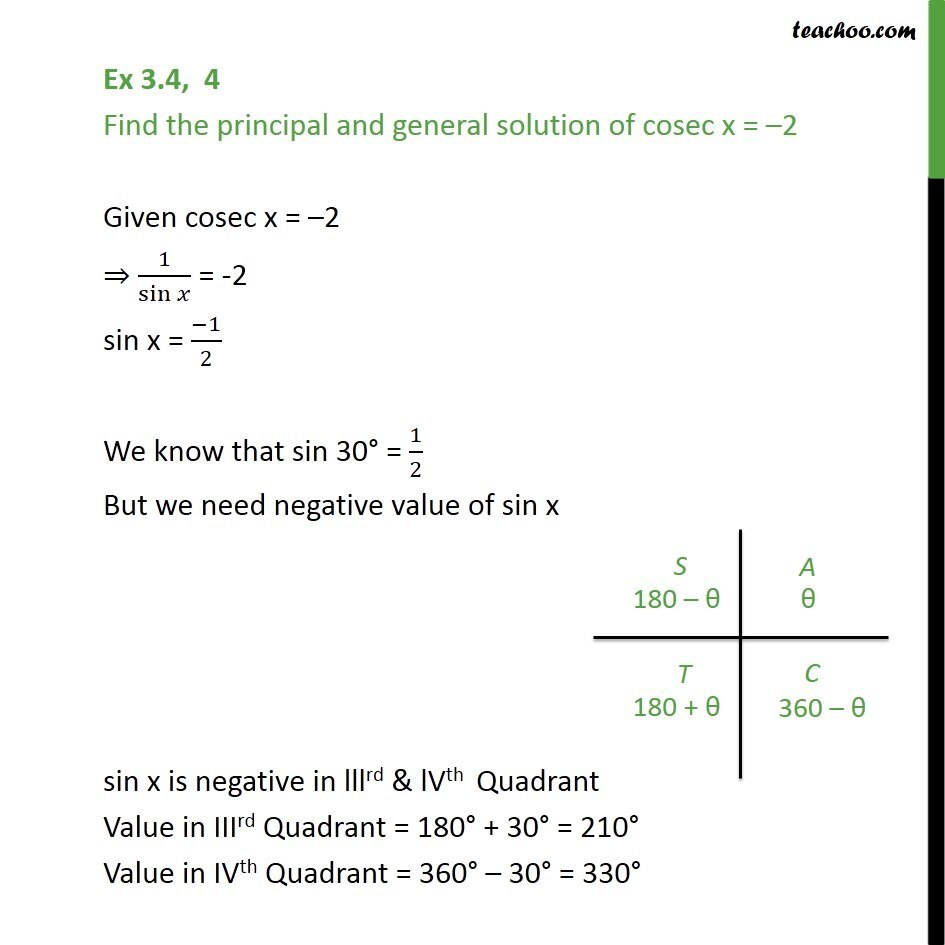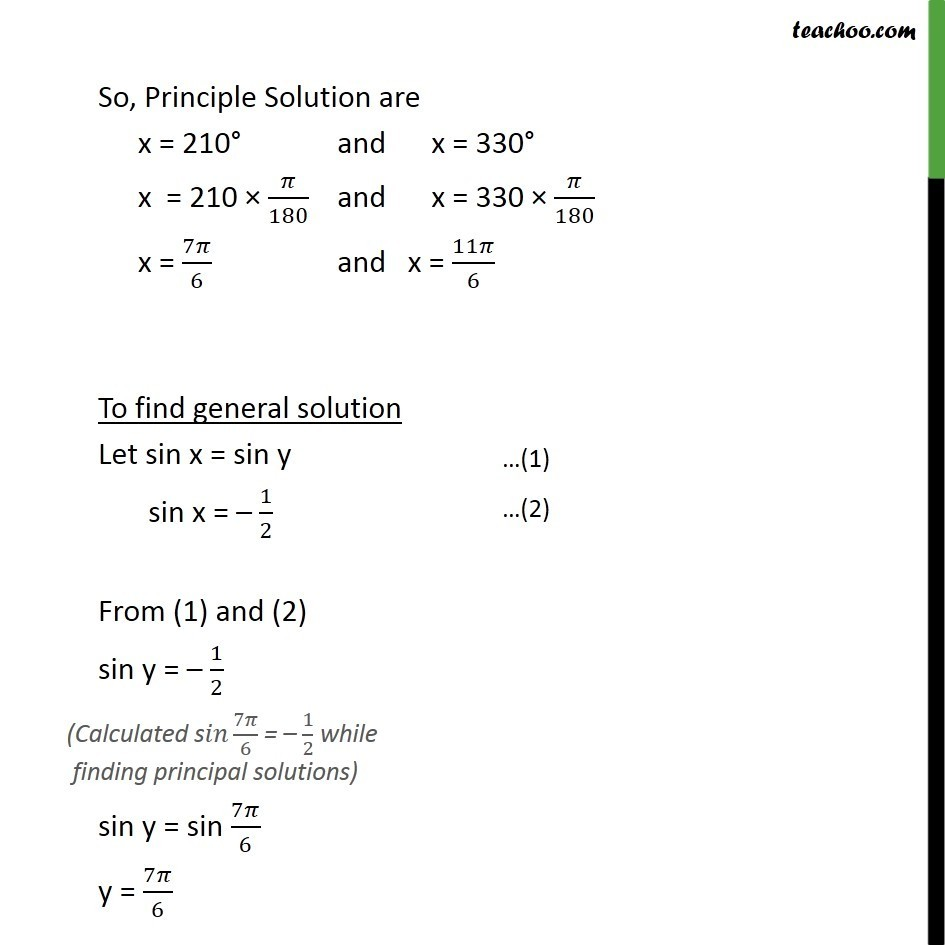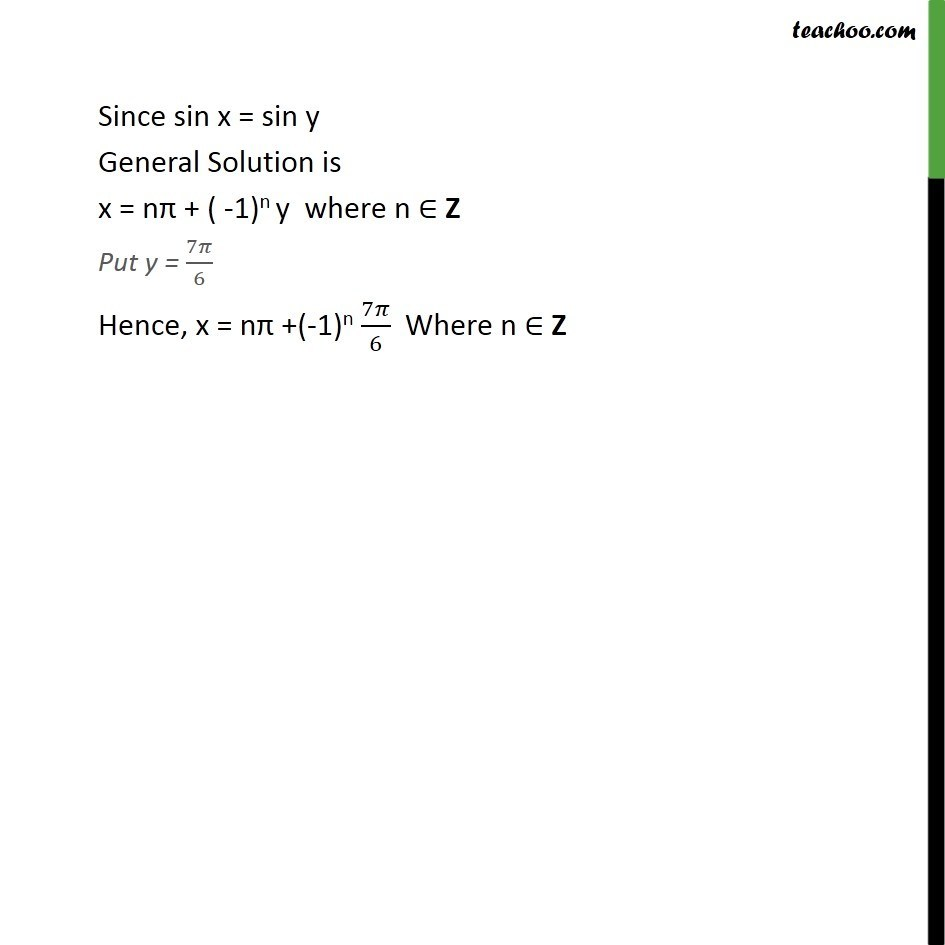1. Class 11
2. Important Question for exams Class 11
3. Chapter 3 Class 11 Trigonometric Functions

Transcript

Ex 3.4, 4 Find the principal and general solution of cosec x = 2 Given cosec x = 2 1/sin = -2 sin x = ( 1)/2 We know that sin 30 = 1/2 But we need negative value of sin x sin x is negative in lllrd & lVth Quadrant Value in IIIrd Quadrant = 180 + 30 = 210 Value in IVth Quadrant = 360 30 = 330 So, Principle Solution are x = 210 and x = 330 x = 210 /180 and x = 330 /180 x = 7 /6 and x = 11 /6 To find general solution Let sin x = sin y sin x = 1/2 From (1) and (2) sin y = 1/2 sin y = sin 7 /6 y = 7 /6 Since sin x = sin y General Solution is x = n + ( -1)n y where n Z Put y = 7 /6 Hence, x = n +(-1)n 7 /6 Where n Z

Chapter 3 Class 11 Trigonometric Functions

Class 11
Important Question for exams Class 11